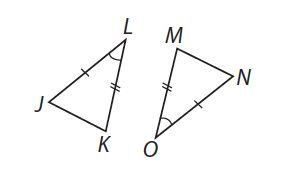# SAT Math Multiple Choice Question 962: Answer and Explanation

### Test Information

Question: 962

14.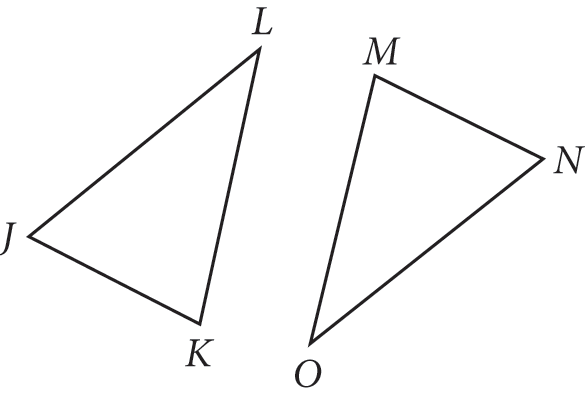In the diagram above,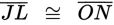and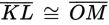. Confirmation of which of the following facts would be sufficient to prove that thetwo triangles are congruent?

• A.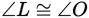• B.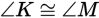• C.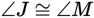• D.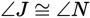Explanation:

A

Difficulty: Easy

Category: Additional Topics in Math / Geometry

Strategic Advice: Think about the options for proving that two triangles are congruent. Then, find the choice that satisfies one of those options.

Getting to the Answer: You're given two congruent pairs of sides, so your options are SSS and SAS. A quick scan of the choices shows that none of them provides congruence for the missing sides, so SSS is out. In order to have SAS, you need to know that the angles between the two congruent sides are congruent. The middle angles in this case are ∠L and ∠O and (as shown below), so the answer must be (A).